### 背景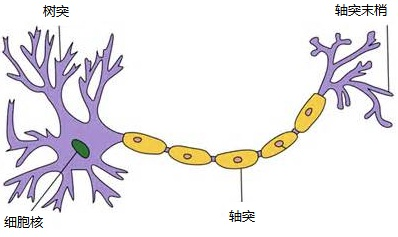1. 1949年心理学家Hebb提出了Hebb学习率，认为人脑神经细胞的突触（也就是连接）上的强度上可以变化的。于是计算科学家们开始考虑用调整权值的方法来让机器学习。这为后面的学习算法奠定了基础。
2. 1958年，计算科学家Rosenblatt提出了由两层神经元组成的神经网络。他给它起了一个名字–感知器（ Perceptron ）
3. 1986年，Rumelhar和Hinton等人提出了反向传播（ Backpropagation ，BP）算法，这是最著名的一个神经网络算法。

### 组成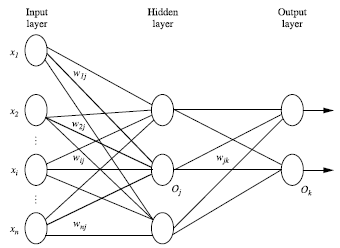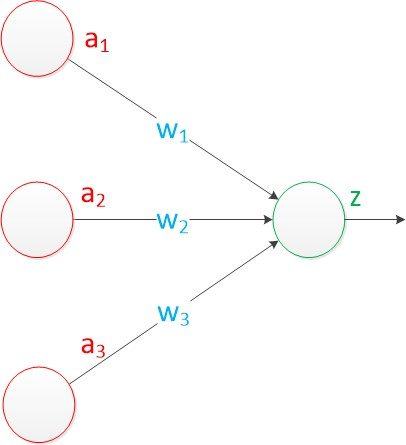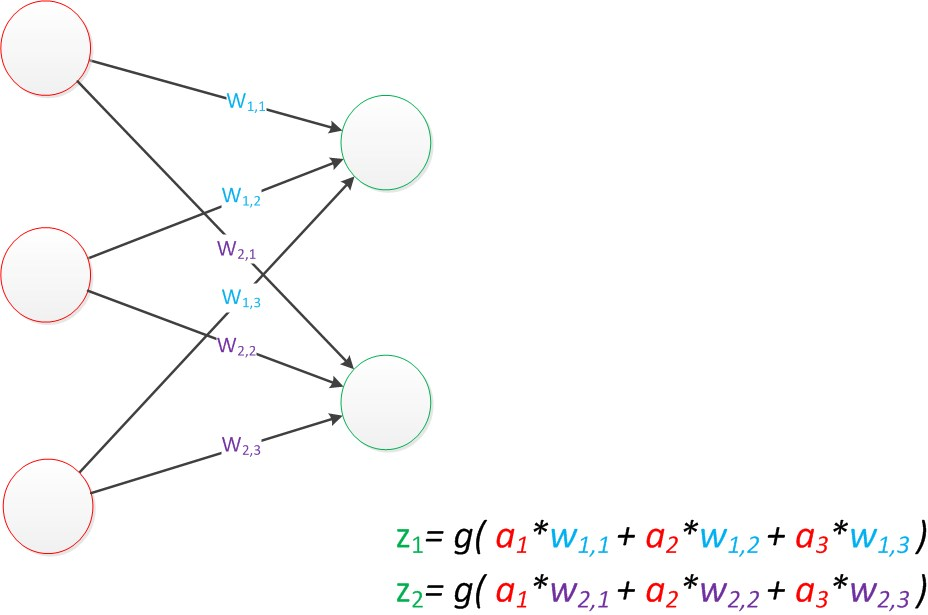### 设计

1. 如果$A=a_0$, 那么代表$a_0$的单元值就取1，其他取0，[1, 0, 0]
2. 如果$A=a_1$, 那么代表$a_1$的单元值就取1，其他取0，[0, 1, 0]
3. 如果$A=a_2$, 那么代表$a_2$的单元值就取1，其他取0，[0, 0, 1]

### 交叉验证### BP算法

BP 算法 (BackPropagation )是多层神经网络的训练一个核心的算法。目的是更新每个连接点的权重，从而减小预测值(predicted value )与真实值 ( target value )之间的差距。输入一条训练数据就会更新一次权重，反方向（从输出层=>隐藏层=>输入层）来以最小化误差（error）来更新权重（weitht）。

BP算法有2个过程，前向传播和反向传播，后者是关键。我们以下图为例，分析BP算法的整个过程。#### 前向传播

$$I_j = \sum_{i}w_{i,j}O_{i} + \theta_{j}$$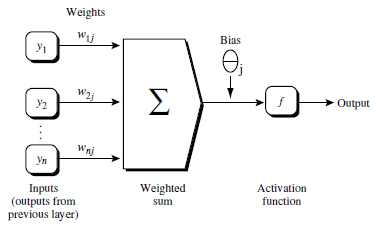$$O_{j} = \frac{1}{1+e^{-I_j}}$$

#### 反向传播

$$Err_{j} = O_{j}(1-O_{j})(T_{j}-O_{j})$$

$$Err_{j} = O_{j}(1-O_{j})\sum_{k}Err_{k}w_{j,k}$$

$$\Delta w_{i,j} = (l)Err_{j}O_{i}$$

$$w_{i,j} = w_{i,j} + \Delta w_{i,j}$$

$$\Delta\theta{j} = (l)Err_{j}$$

$$\theta{j} = \theta_{j} + \Delta\theta_{j}$$

#### 终止条件

1. 权重的更新低于某个阈值，这个阈值是可以人工指定的；
2. 预测的错误率低于某个阈值；
3. 达到预设一定的循环次数。

#### S型函数（sigmod）

1. 双曲函数（tanh
2. 逻辑函数（logistic function
Sigmod函数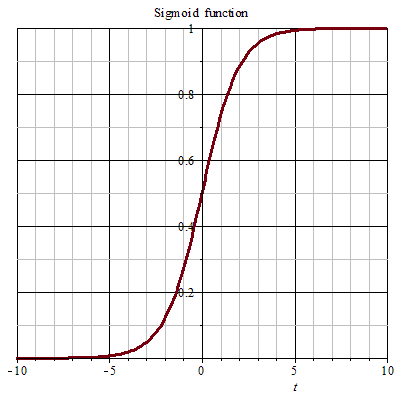S 曲线函数可以将一个数值转为值域在 0 到 1 之间，广义上S 函数是满足y值在某个值域范围内渐变的一条曲线。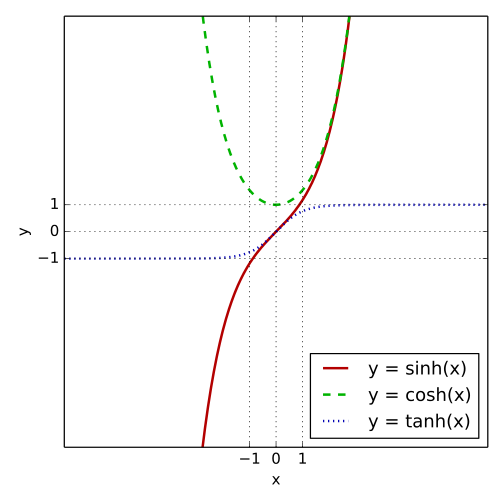$$tanhx = \frac{sinhx}{coshx}$$

$$\frac{d}{dx}tanhx = 1 - tanh^2x =sech^2x = \frac{1}{cosh^2x}$$$$f(x) = \frac{1}{1 + e^{-x}}$$

$$\frac{d}{dx} = \frac{e^x}{(1+e^x)^2} = f(x)(1 - f(x))$$

#### BP算法举例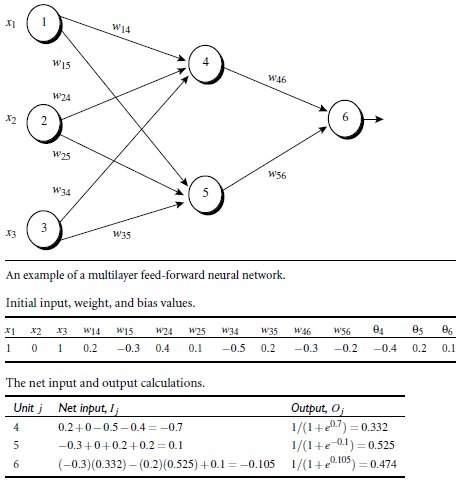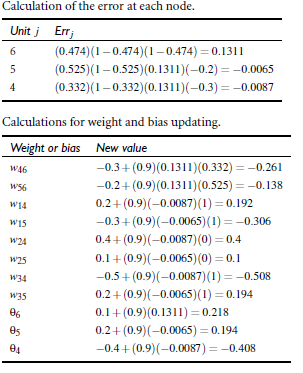### 代码实例（手写数字识别）

#### 网络函数#### 手写数字图片识别------ 本文结束------